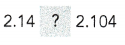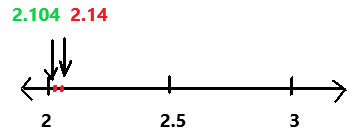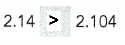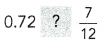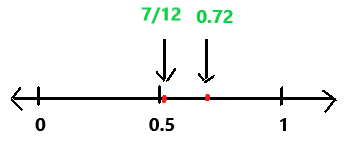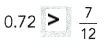# Math in Focus Grade 6 Chapter 2 Answer Key Negative Numbers and the Number Line

Go through the Math in Focus Grade 6 Workbook Answer Key Chapter 2 Negative Numbers and the Number Line to finish your assignments.

## Math in Focus Grade 6 Course 1 A Chapter 2 Answer Key Negative Numbers and the Number Line

### Math in Focus Grade 6 Chapter 2 Quick Check Answer Key

Draw a horizontal number line to represent each set of numbers.

Question 1.
Odd numbers from 20 to 30
Odd numbers from 20 to 30 are 21, 23, 25, 27, 29.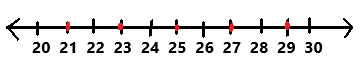Explanation:
Odd numbers from 20 to 30 are 21, 23, 25, 27, 29 are represented in the above horizontal number line. On a horizontal number line, the numbers become greater as you move to the right, and less as you move to the left.

Question 2.
Mixed numbers from 3 to 5, with an interval of $$\frac{1}{6}$$ between each pair of mixed numbers
Mixed numbers from 3 to 5, with an interval of $$\frac{1}{6}$$ between each pair of mixed numbers are 3, 3(1/6), 3(2/6), 3(3/6), 3(4/6), 3(5/6), 4, 4(1/6), 4(2/6), 4(3/6), 4(4/6), 4(5/6), 5.

Question 3.
Decimals between 8.0 and 10.0, with an interval of 0.25 between each pair of decimals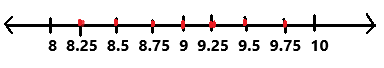Explanation:
Decimals between 8.0 and 10.0, with an interval of 0.25 between each pair of decimals8.25, 8.5, 8.75, 9, 9.25, 9.5, 9.75 are represented in the above horizontal number line. On a horizontal number line, the numbers become greater as you move to the right, and less as you move to the left.

Compare each pair of numbers using > or <. Use a number line to help you.

Question 4.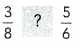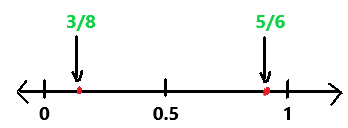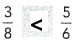Explanation:
The given numbers are 3/8 and 5/6. Here we have to compare the pair of numbers. On a horizontal number line, the numbers become greater as you move to the right, and less as you move to the left as we can observe in the above image. After comparing numbers on the number line 3/8 is less than 5/6.

Question 5.